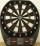# Difference of two number

The difference of two numbers is 20. They are positive integers greater than zero. The first number raised to one-half equals the second number. Determine the two numbers.

Result

a =  25
b =  5

#### Solution:Checkout calculation with our calculator of quadratic equations.Leave us a comment of example and its solution (i.e. if it is still somewhat unclear...):

Showing 0 comments:Be the first to comment!#### To solve this example are needed these knowledge from mathematics:

Looking for help with calculating roots of a quadratic equation? Do you have a linear equation or system of equations and looking for its solution? Or do you have quadratic equation?

## Next similar examples:

1. Find twoFind two consecutive natural numbers whose product is 1 larger than their sum. Searched numbers expressed by a fraction whose numerator is the difference between these numbers and the denominator is their sum.
2. TargetPeter, Martin and Jirka were fire in a special target, which had only three fields with values of 12, 18 and 30 points. All boys were firing with the same number of arrows and all the arrows hit the target, and the results of every two boys differed in one
3. Ball gameRichard, Denis and Denise together scored 932 goals. Denis scored 4 goals over Denise but Denis scored 24 goals less than Richard. Determine the number of goals for each player.
4. Theorem proveWe want to prove the sentense: If the natural number n is divisible by six, then n is divisible by three. From what assumption we started?
5. Three workshopsThere are 2743 people working in three workshops. In the second workshop works 140 people more than in the first and in third works 4.2 times more than the second one. How many people work in each workshop?
6. LegsCancer has 5 pairs of legs. The insect has 6 legs. 60 animals have a total of 500 legs. How much more are cancers than insects?
7. ChildrenThe group has 42 children. There are 4 more boys than girls. How many boys and girls are in the group?
8. Men, women and childrenOn the trip went men, women and children in the ratio 2:3:5 by bus. Children pay 60 crowns and adults 150. How many women were on the bus when a bus was paid 4,200 crowns?
9. Linsys2Solve two equations with two unknowns: 400x+120y=147.2 350x+200y=144
10. EquationEquation ? has one root x1 = 8. Determine the coefficient b and the second root x2.
11. Solve 3Solve quadratic equation: (6n+1) (4n-1) = 3n2
12. Elimination methodSolve system of linear equations by elimination method: 5/2x + 3/5y= 4/15 1/2x + 2/5y= 2/15
13. VariableFind variable P: PP plus P x P plus P = 160
14. Quadratic equationFind the roots of the quadratic equation: 3x2-4x + (-4) = 0.
15. DiscriminantDetermine the discriminant of the equation: ?
16. Three unknownsSolve the system of linear equations with three unknowns: A + B + C = 14 B - A - C = 4 2A - B + C = 0
17. RootsDetermine the quadratic equation absolute coefficient q, that the equation has a real double root and the root x calculate: ?